Definitions of Square Dance Calls and Concepts
Exchange The Triangle [C3A]

Index -->  Plus  |  A1  |  A2  |  C1  |  C2  |  C3A  |  C3B  |  C4  |  NOL  |
Definitions (Text Only) -->  Plus  |  A1  |  A2  |  C1  |  C2  |  C3A  |  C3B  |  C4  |  NOL  |
 Find call:

 \$B8@8l(BTwin Triangles (1 \$BBP\$N(B Triangle) \$B\$+\$i!%(B

\$B\$N(B Triangle \$B\$X(B Circulate \$B\$7\$^\$9!%(B (Apex-to-Apex Triangles \$B\$+\$i\$N(B Circulate \$B\$O(B Interlocked Triangle Circulate \$B\$H\$J\$j(B, Base-to-Base Triangles \$B\$+\$i\$N(B Circulate \$B\$OExchange The Triangle \$B\$O(B 3 \$B\$D\$N%Q!<%H\$KJ,\$1\$i\$l\$^\$9!%(B

Apex-to-Apex Wave-Based Triangles \$B\$+\$i(B (Very Centers \$B\$,%j!<%I(B):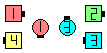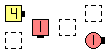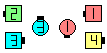Exchange The Triangles\$B\$NA0(B \$B:8\$N(B Triangle 1/3 \$B\$N8e(B... \$B8e(B

Base-to-Base Tandem-Based Triangles \$B\$+\$i(B (Trailing Centers \$B\$,%j!<%I(B):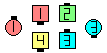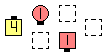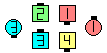Exchange The Triangles\$B\$NA0(B \$B:8\$N(B Triangle 1/3 \$B\$N8e(B... \$B8e(B

\$BCm\$N(B formation \$B\$N30B&\$r(B Circulate \$B\$7\$^\$9!%\$=\$7\$F%*%j%8%J%k\$N(B formation \$B\$N(B footprint (\$BLu

Exchange The 3 By 1 Triangle [NOL] (Very Centers \$B\$,%j!<%I(B)

(Ciculate \$B\$r9g7W(B 4 \$B2s9T\$\$\$^\$9(B).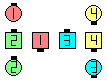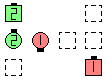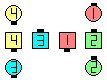Exchange The 3 By 1 Triangles\$B\$NA0(B \$B:8\$N(B Triangle 1/4 \$B\$N8e(B... \$B8e(B

Exchange The 3 By 1 Triangles [NOL] (Very Centers \$B\$,%j!<%I(B)

(Ciculate \$B\$r9g7W(B 4 \$B2s9T\$\$\$^\$9(B).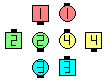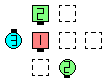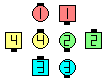Exchange The 3 By 1 Triangles\$B\$NA0(B \$B:8\$N(B Triangle 1/4 \$B\$N8e(B... \$B8e(B

\$BExchange The Box [C3A].CALLERLAB definition for Exchange The TriangleChoreography for Exchange The TriangleComments? Questions? Suggestions?

https://www.ceder.net/def/exchtriangle.php?level=C3A&language=japan
19-November-2019 20:24:16